Get instant live expert help with Excel or Google Sheets“My Excelchat expert helped me in less than 20 minutes, saving me what would have been 5 hours of work!”

#### Post your problem and you’ll get expert help in seconds.

Your message must be at least 40 characters
Our professional experts are available now. Your privacy is guaranteed.

# Excel WEEKDAY Function

We can use the Excel WEEKDAY function to extract a weekday as a numeric value. This is an inbuilt function that is great for scheduling. In straightforward steps, we will walk through the process of using this function.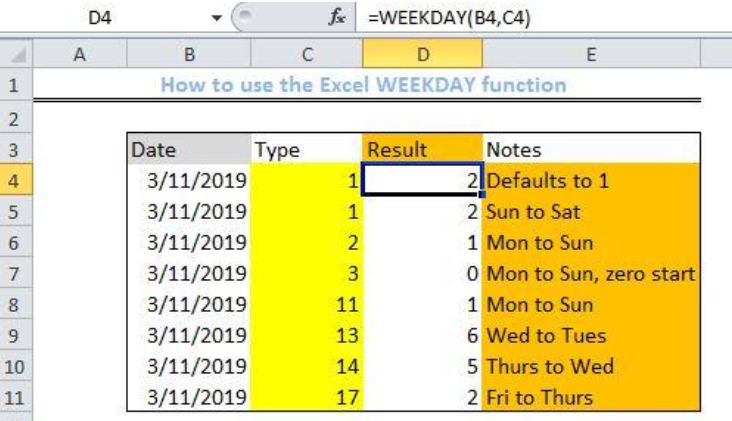Figure 1 – Result of using the Excel WEEKDAY function

## General Formula

`=WEEKDAY(serial_num,[return_type])`

• Serial_num: represents the date whose day of the week we want to get
• Return_type: a number representing the mapping scheme. By default, the value is 1

## Formula

`= WEEKDAY(B4,C4)`

## Setting up the Data

• We will set up our list of Dates in column B
• Column C will contain our list of specific mapping types
• Column D is where we will insert the formula and arrive at the Result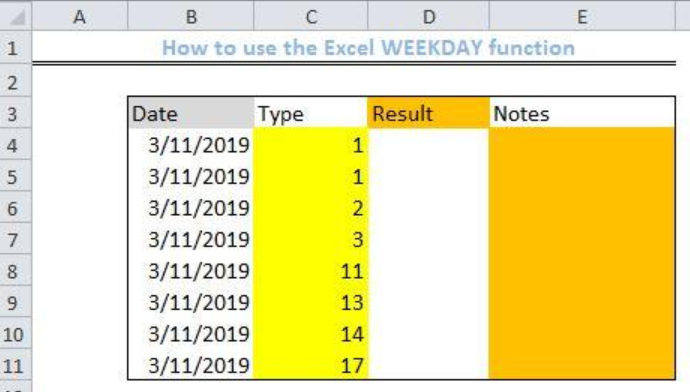Figure 2- Setting up the Data

## Applying the WEEKDAY function

• We will click on Cell D4
• In this cell, we will place the formula below
`=WEEKDAY (B4,C4)`
• We will press the enter key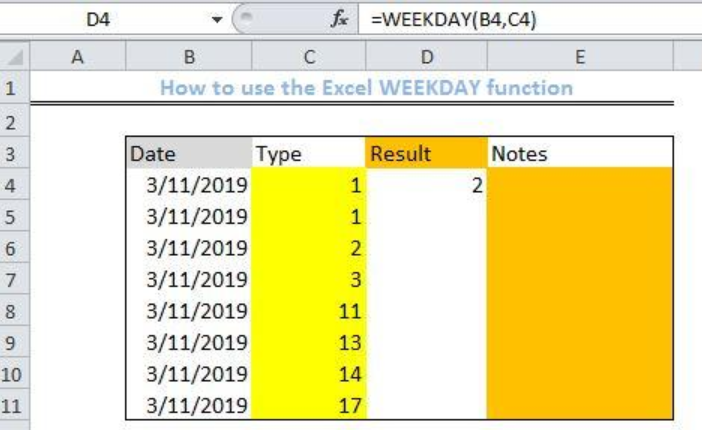Figure 3 – Applying the Excel WEEKDAY function

• We will select the Cell D4 again
• We will double click on the fill handle tool which is the small plus sign you see at the bottom right of Cell D4. Select and drag down to copy the formula to the other cells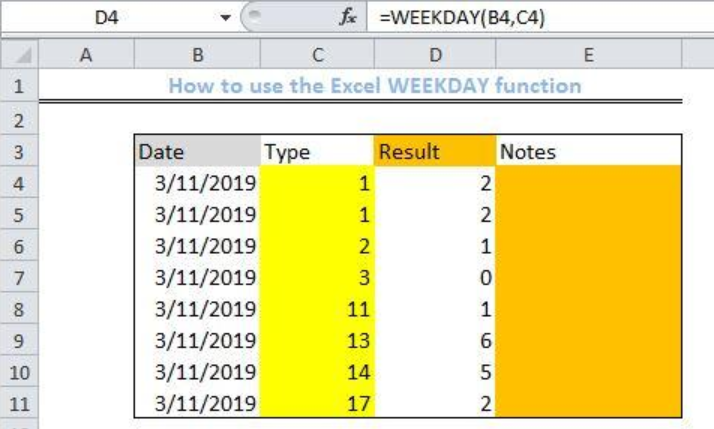Figure 4 – Result of using the Excel WEEKDAY function

## Explanation

We use the WEEKDAY function to arrive at a number (between 1 and 7) that represents the day of the week. However, the mapping type would determine the outcome. By default, 1 represents Sunday, while 7 represents Saturday. But, changing the mapping type will change the value of the outcome.

The default mapping type would result in the table below: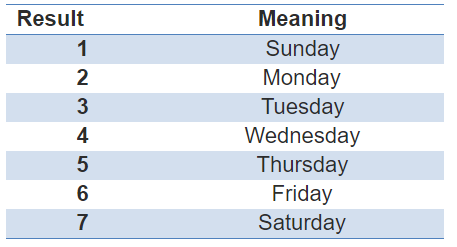Table 1 – Default mapping type, result, and meaning

The WEEKDAY function supports different mapping schemes. By changing the kind of argument, we will change the outcome. The table below shows the results and the associated mapping scheme.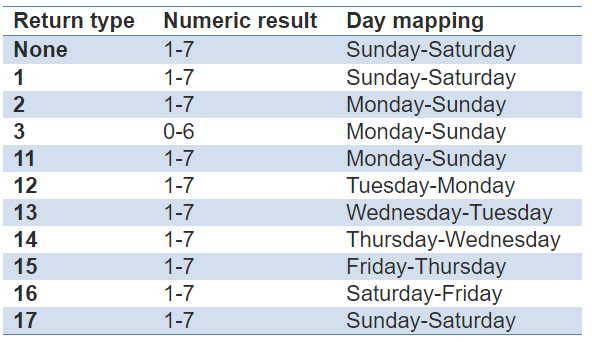Table 2 – Different kinds of day mapping types

## Notes

• The WEEKDAY function will return with a numeric value even if the date parameter is blank.
• We can also use the WEEKDAY function to perform other operations such as creating custom weekdays or the Gantt chart.

## Instant Connection to an Expert through our Excelchat Service

Most of the time, the problem you will need to solve will be more complex than a simple application of a formula or function. If you want to save hours of research and frustration, try our live Excelchat service! Our Excel Experts are available 24/7 to answer any Excel question you may have. We guarantee a connection within 30 seconds and a customized solution within 20 minutes.

### Did this post not answer your question? Get a solution from connecting with the expert.Another blog reader asked this question today on Excelchat:
Solution examplesneed help with the different sheets and formula IF(A4=B4,0,NETWORKDAYS(A4+1,B4,\$E\$9:\$E\$42) +0.5*(INT((+B4-A4+1)/7)+IF(WEEKDAY(A4+1)+MOD (B4-A4-1,7)>=7,1,0)))
Solved by O. D. in 11 mins## Subscribe to Excelchat.coAnother blog reader asked this question today on Excelchat: